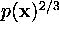Next: Relation to principal curves. Up: Mathematical characterizations Previous: Mathematical characterizations

#### Relation to K-means clustering.

The cost function of the SOM, Equation 7, closely resembles Equation 1, which the K-means clustering algorithm tries to minimize. The difference is that in the SOM the distance of each input from all of the reference vectors instead of just the closest one is taken into account, weighted by the neighborhood kernel h. Thus, the SOM functions as a conventional clustering algorithm if the width of the neighborhood kernel is zero.

The close relation between the SOM and the K-means clustering algorithm also hints at why the self-organized map follows rather closely the distribution of the data set in the input space: it is known for vector quantization that the density of the reference vectors approximates the density of the input vectors for high-dimensional data [Kohonen, 1995c, Zador, 1982], and K-means is essentially equivalent to vector quantization. In fact, an expression for the density of the reference vectors of the SOM has been derived in the one-dimensional case [Ritter, 1991]; in the limit of a very wide neighborhood and a large number of reference vectors the density is proportional to, whereis the probability density function of the input data.

Note: Although the K-means clustering algorithm and the SOM are very closely related, the best ways of using them in data mining are probably different. Whereas in the K-means clustering algorithm the number K of clusters should be chosen according to the number of clusters there are in the data, in the SOM the number of reference vectors can be chosen to be much larger, irrespective of the number of clusters. The cluster structures will become visible on the special displays that were discussed in Section 6.4.2.